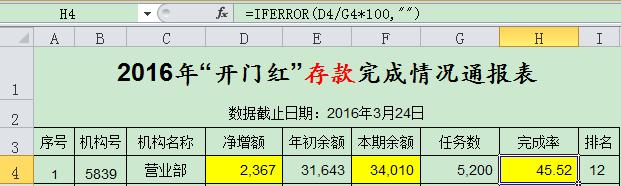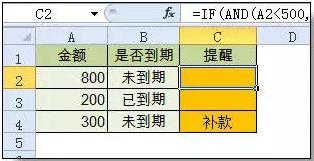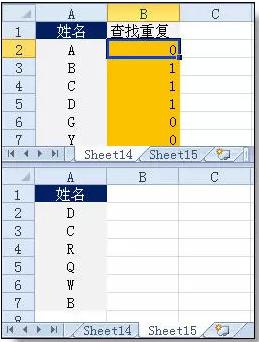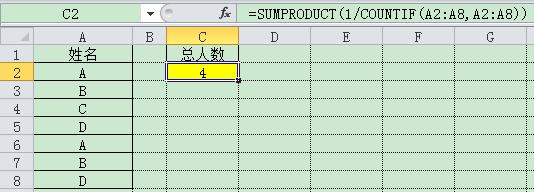• 2021-06-30 00:39:53

Excel是工作中最常用的电脑办公工具之一。下面是学习啦小编为您精心整理的最常用的Excel函数公式大全。

篇一

零、随机数

1、随机数函数：

=RAND()

首先介绍一下如何用RAND()函数来生成随机数(同时返回多个值时是不重复的)。RAND()函数返回的随机数字的范围是大于0小于1。因此，也可以用它做基础来生成给定范围内的随机数字。

生成制定范围的随机数方法是这样的，假设给定数字范围最小是A，最大是B，公式是：=A+RAND()*(B-A)。

举例来说，要生成大于60小于100的随机数字，因为(100-60)*RAND()返回结果是0到40之间，加上范围的下限60就返回了60到100之间的数字，即=60+(100-60)*RAND()。

2、随机整数

=RANDBETWEEN(整数，整数)

如：=RANDBETWEEN(2,50)，即随机生成2~50之间的任意一个整数。

上面RAND()函数返回的0到1之间的随机小数，如果要生成随机整数的话就需要用RANDBETWEEN()函数了，如下图该函数生成大于等于1小于等于100的随机整数。

这个函数的语法是这样的：=RANDBETWEEN(范围下限整数，范围上限整数)，结果返回包含上下限在内的整数。注意：上限和下限也可以不是整数，并且可以是负数。

篇二

求和公式

1、隔列求和

公式：H3

=SUMIF($A$2:$G$2,H$2,A3:G3) =SUMPRODUCT((MOD(COLUMN(B3:G3),2)=0)*B3:G3) 说明：如果标题行没有规则用第2个公式 2、单条件求和 公式：F2 =SUMIF(A:A,E2,C:C) 说明：SUMIF函数的基本用法 3、单条件模糊求和 公式：详见下图 说明：如果需要进行模糊求和，就需要掌握通配符的使用，其中星号是表示任意多个字符，如"*A*"就表示a前和后有任意多个字符，即包含A。 4、多条件模糊求和 公式：C11 =SUMIFS(C2:C7,A2:A7,A11&"*",B2:B7,B11) 说明：在sumifs中可以使用通配符* 5、多表相同位置求和 公式：b2 =SUM(Sheet1:Sheet19!B2) 说明：在表中间删除或添加表后，公式结果会自动更新。 6、按日期和产品求和 公式：F2 =SUMPRODUCT((MONTH($A$2:$A$25)=F$1)*($B$2:$B$25=$E2)*$C$2:$C$25) 说明：SUMPRODUCT可以完成多条件求和 篇三 字符串处理公式 1、多单元格字符串合并 公式：c2 =PHONETIC(A2:A7) 说明：Phonetic函数只能对字符型内容合并，数字不可以。 2、截取除后3位之外的部分 公式： =LEFT(D1,LEN(D1)-3) 说明：LEN计算出总长度,LEFT从左边截总长度-3个 3、截取-前的部分 公式:B2 =Left(A1,FIND("-",A1)-1) 说明：用FIND函数查找位置，用LEFT截取。 4、截取字符串中任一段的公式 公式:B1 =TRIM(MID(SUBSTITUTE($A1," ",REPT(" ",20)),20,20))

说明:公式是利用强插N个空字符的方式进行截取

参加试验检测考试加微信:13527870418购买开通微试验题库

5、字符串查找

公式：B2

=IF(COUNT(FIND("河南",A2))=0,"否","是")

说明: FIND查找成功，返回字符的位置，否则返回错误值，而COUNT可以统计出数字的个数，这里可以用来判断查找是否成功。

6、字符串查找一对多

公式：B2

=IF(COUNT(FIND({"辽宁","黑龙江","吉林"},A2))=0,"其他","东北")

说明：设置FIND第一个参数为常量数组，用COUNT函数统计FIND查找结果

更多相关内容
• ## MySQL 一对多查询

千次阅读 2021-01-18 18:49:43
导语这次要实现的是一对多查询，使用 MySQL 的 group_concat 函数实现。group_concat简单来说，这个函数的作用就是连接多个字段，函数的具体详解可以看这篇文章。数据表首先我们先建立两个表CREATE TABLE student ...

导语

这次要实现的是一对多查询，使用 MySQL 的 group_concat 函数实现。

group_concat

简单来说，这个函数的作用就是连接多个字段，函数的具体详解可以看这篇文章。

数据表

首先我们先建立两个表

CREATE TABLE student (

id int(11) NOT NULL AUTO_INCREMENT,

name char(10) NOT NULL,

PRIMARY KEY (id)

) ENGINE=MyISAM AUTO_INCREMENT=3 DEFAULT CHARSET=utf8;

-- ----------------------------

-- Records of student

-- ----------------------------

INSERT INTO student VALUES ('1', 'tom');

INSERT INTO student VALUES ('2', 'jerry');

CREATE TABLE course (

id int(11) NOT NULL AUTO_INCREMENT,

s_id int(11) NOT NULL,

c_name char(10) NOT NULL,

PRIMARY KEY (id)

) ENGINE=MyISAM AUTO_INCREMENT=6 DEFAULT CHARSET=utf8;

-- ----------------------------

-- Records of course

-- ----------------------------

INSERT INTO course VALUES ('1', '1', '语文');

INSERT INTO course VALUES ('2', '1', '数学');

INSERT INTO course VALUES ('3', '2', '英语');

INSERT INTO course VALUES ('4', '2', '体育');

INSERT INTO course VALUES ('5', '2', '美术');

实例

如果我们用平时使用的 SQL 查询

SELECT s.name,c.c_name FROM student AS s LEFT JOIN course AS c ON c.s_id = s.id;

查询出的结果是查询结果

下面用 group_concat 函数查询

SELECT s.name,(SELECT group_concat(course.c_name) FROM course WHERE course.s_id = s.id) FROM student AS s;

此时查询的结果查询结果

展开全文• 今天和大家分享组常用函数公式的使用方法，用心掌握这些函数，工作效率会大大提升。欢迎转发给更有需要的人。、相关概念()函数语法由函数名+括号+参数组成例： 求和函数：SUM(A1,B2,…) 。参数与参数之 间用...

今天和大家分享一组常用函数公式的使用方法，用心掌握这些函数，工作效率会大大提升。欢迎转发给更多有需要的人。

一、相关概念

(一)函数语法

由函数名+括号+参数组成

例： 求和函数：SUM(A1,B2,…) 。参数与参数之 间用逗号“,”隔开

(二)运算符

1. 公式运算符：加(+)、减(-)、乘(*)、除(/)、百分号(%)、乘幂(＾)

2. 比较运算符：大与(>)、小于(=) 、不等于(<>)

3. 引用运算符：区域运算符(：)、联合运算符(,)

(三)单元格的相对引用与绝对引用

例： A1

$A1 锁定第A列 A$1 锁定第1行

$A$1 锁定第A列与第1行

二、常用函数

(一)数学函数

1. 求和 =SUM(数值1,数值2,……)

2. 条件求和 =SUMIF(查找的范围,条件(即对象),要求和的范围)

例：(1)=SUMIF(A1:A4,”>=200”,B1:B4)

函数意思：对第A1栏至A4栏中，大于等于200的数值对应的第B1列至B4列中数值求和

(2)=SUMIF(A1:A4,”<300”,C1:C4)

函数意思：对第A1栏至A4栏中，小于300的数值对应的第C1栏至C4栏中数值求和

3. 求个数 =COUNT(数值1,数值2,……)

例：(1) =COUNT(A1:A4) 函数意思：第A1栏至A4栏求个数

(2) =COUNT(A1:C4) 函数意思：第A1栏至C4栏求个数

4. 条件求个数 =COUNTIF(范围,条件)

例：(1) =COUNTIF(A1:A4，”<>200”)

函数意思：第A1栏至A4栏中不等于200的栏求个数

(2) =COUNTIF(A1:C4,”>=1000”)

函数意思：第A1栏至C4栏中大于等1000的栏求个数

5. 求算术平均数 =AVERAGE(数值1,数值2,……)

例：(1) =AVERAGE(A1,B2)

(2) =AVERAGE(A1:A4)

6. 四舍五入函数 =ROUND(数值,保留的小数位数)

7. 排位函数 =RANK(数值,范围,序别) 1-升序 0-降序

例：(1) =RANK(A1,A1:A4,1)

函数意思：第A1栏在A1栏至A4栏中按升序排序，返回排名值。

(2) =RANK(A1,A1:A4,0)

函数意思：第A1栏在A1栏至A4栏中按降序排序，返回排名值。

8. 乘积函数 =PRODUCT(数值1,数值2,……)

9. 取绝对值 =ABS(数字)

10. 取整 =INT(数字)

(二)逻辑函数

条件函数：=IF(条件,执行条件,执行条件假)—可执行七层嵌套

例： A1=5 A2=3 A3=6 A4=7

=IF(A1>A2,1,0)=1(真)

=IF(A1

=IF(A1>A2,IF(A3>A4,8,9),1)=9

(三)文本函数

1. 截取函数： =LEFT(文本,截取长度)—从左边截取

=RIGHT(文本,截取长度)—从右边截取

=MID(文本,开始位,截取长度)—从中间截取

2. 计算字符长度：LEN(文本)—文本的长度

3. 合并字符函数：CONCATENATE(文本1,文本2,…)

4. 在字符串中查找特定字符：FIND(文本,范围,数值)—数值表示查找第几个

5. 比较两个字符是否完全相符：EXACT(文本1,文本2)

=EXACT(“a”,“a”)=TRUE

=EXACT(“a”,“ab”)=FALSE

=EXACT(“a”,“A”)=FALSE

6. 将数值转化为文本：TEXT(数值,参数) )—参数一般为0

7. 将数值型字符转换为数值：VALUE(数值文本)

(四)判断公式

1. 把公式产生的错误显示为空

公式：C2=IFERROR(A2/B2,””)

说明：如果是错误值则显示为空，否则正常显示。2. IF多条件判断返回值

公式：C2=IF(AND(A2<500,B2=”未到期”),”补款”,””)

说明：两个条件同时成立用AND，任一个成立用OR函数。(五)统计公式

1. 统计两个表格重复的内容

公式：B2=COUNTIF(Sheet15!A:A,A2)

说明：如果返回值大于0说明在另一个表中存在，0则不存在。2. 统计不重复的总人数

公式：C2=SUMPRODUCT(1/COUNTIF(A2:A8,A2:A8))

说明：用COUNTIF统计出每人的出现次数，用1除的方式把出现次数变成分母，然后相加。(六)求和公式

1. 隔列求和

• ## Excel2013函数公式大全（一）

万次阅读 多人点赞 2019-03-06 18:05:22
1、函数的定义与作用 ...3、写函数公式时的注意事项 怎么写函数？ 怎么补全函数？ 怎么填写参数？ 怎么复制填充公式？ 4、excel常用数学运算符 算术运算符 含义 +（加号） 加法 -（减号...
• 更新说明：为满足部分考研学子的需求，本次...说到三角函数公式，不管是高考也好还是考研也好，都逃不脱高中必修四“三角函数”部分，作为复习过来人，我将通过口诀以及图解的方式来帮助更的同学来理解公式(以下...
• ## Excel函数公式大全以及用法

万次阅读 多人点赞 2018-09-07 10:25:23
首先得说明下excel如何输入函数公式的方法方法是：单击a4单元格（a4单元格的位置是第A列，第4行交叉的那个单元格，也即是我们彩色实例的那个位置），在键盘上输入 =a1+a2+a3 ，然后按键盘的enter键（回车键）即可...
• 《Excel 2010函数公式》包括282疑难案例，每个疑难都包含个或个解决方案，并融入了个或个知识点的讲解，在解决问题的同时，引导读者学习公式中的原理和扩展知识。书中还介绍了大量成熟的通用公式，读者...excel
• 三角函数公式及工程应用 起源及发展历史   早期对于三角函数的研究可以追溯到古代。古希腊三角术的奠基人是公元前2世纪的喜帕恰斯。他按照古巴比伦人的做法，将圆周分为360等份（即圆周的弧度为360度......